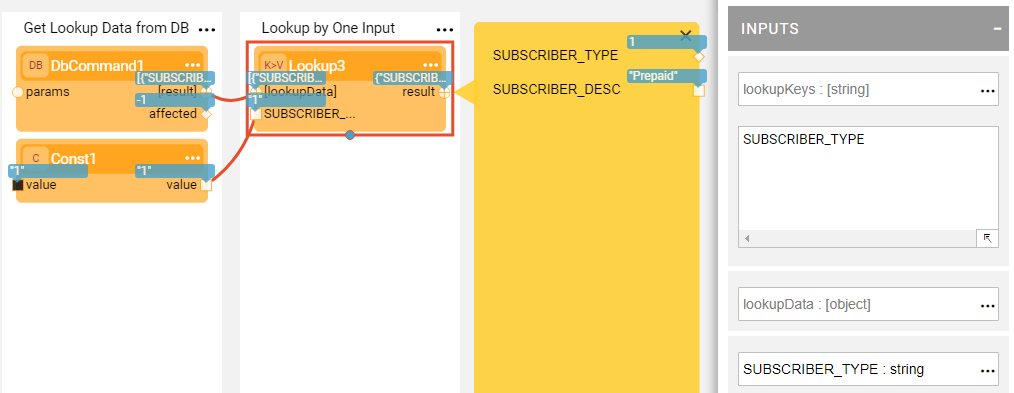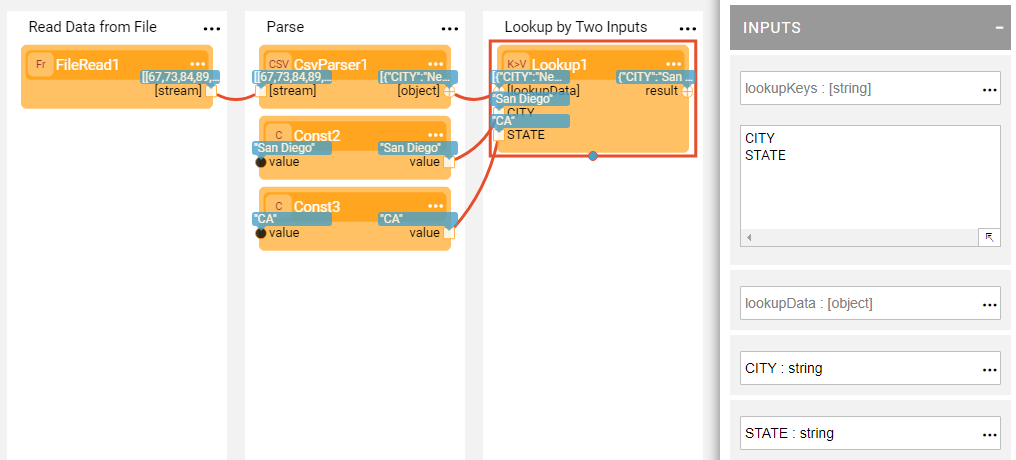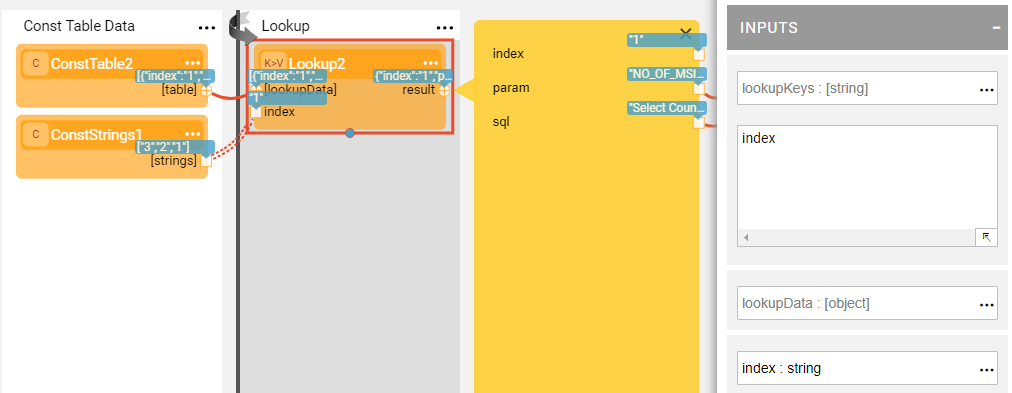X

### Translation or Lookup of Data Using Broadway

Lookup for a data row based on provided input is a common scenario in various population and data transformation flows.

The Broadway Lookup Actor enables performing a data lookup or a data translation from various sources and based on a flexible number of input parameters.

Example 1 - Lookup Data Based on DB Select

When a lookup data is coming from a DB, use the DbCommand Actor in order to select the required data. Then in the lookupKeys input argument of the Lookup Actor, populate the name of the column to be used as an input for a lookup (or data translation). A new port with the same name will be added to the Lookup Actor.

Provide an input for a lookup key and run the flow. Accordingly, the Lookup Actor will return the required data row.Example 2 - Lookup Data Based on File

It is similar to Example 1, however, this time the data is coming from a file rather than from a DB. In this case, use the FileRead Actor in order to read the CSV file and then use the CsvParser to parse it.

This example shows that two lookup keys were defined for retrieving the required data row.Example 3 - Lookup Data Based on Const Table

When the data is constant and is not supposed to change, a ConstTable Actor can be used for storing the lookup data. Also in this case, you need to populate the lookupKeys argument with the name of the ConstTable Actor's column.

This example shows that you can iterate on the lookup by providing different values for the lookup key.### Translation or Lookup of Data Using Broadway

Lookup for a data row based on provided input is a common scenario in various population and data transformation flows.

The Broadway Lookup Actor enables performing a data lookup or a data translation from various sources and based on a flexible number of input parameters.

Example 1 - Lookup Data Based on DB Select

When a lookup data is coming from a DB, use the DbCommand Actor in order to select the required data. Then in the lookupKeys input argument of the Lookup Actor, populate the name of the column to be used as an input for a lookup (or data translation). A new port with the same name will be added to the Lookup Actor.

Provide an input for a lookup key and run the flow. Accordingly, the Lookup Actor will return the required data row.Example 2 - Lookup Data Based on File

It is similar to Example 1, however, this time the data is coming from a file rather than from a DB. In this case, use the FileRead Actor in order to read the CSV file and then use the CsvParser to parse it.

This example shows that two lookup keys were defined for retrieving the required data row.Example 3 - Lookup Data Based on Const Table

When the data is constant and is not supposed to change, a ConstTable Actor can be used for storing the lookup data. Also in this case, you need to populate the lookupKeys argument with the name of the ConstTable Actor's column.

This example shows that you can iterate on the lookup by providing different values for the lookup key.